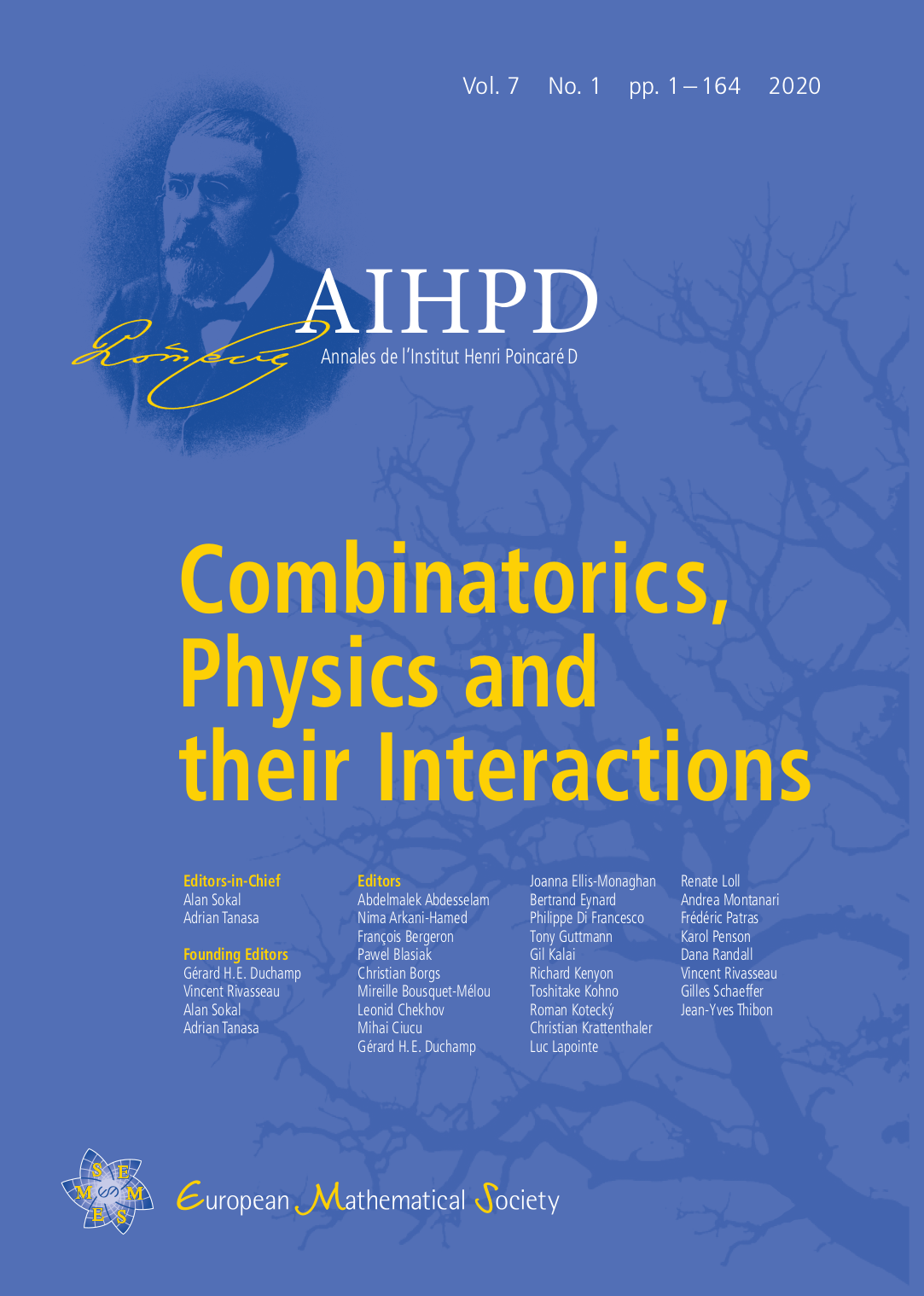AIHPD

# Annales de l’Institut Henri Poincaré D

DOI: 10.4171/AIHPD | ISSN: 2308-5827 | eISSN: 2308-5835The unfolding of new ideas in physics is often tied to the development of new combinatorial methods, and conversely some problems in combinatorics have been successfully attacked using methods inspired by statistical physics or quantum field theory.

The journal is dedicated to publishing high-quality original research articles and survey articles in which combinatorics and physics interact in both directions. Combinatorial papers should be motivated by potential applications to physical phenomena or models, while physics papers should contain some interesting combinatorial development.

Both rigorous mathematical proof and heuristic physical reasoning have a place in this journal, but each must be clearly labeled. Definitions and proofs should be presented with the precision and rigor that are expected in a mathematics journal. Conjectures based on heuristic physical arguments and/or numerical evidence are warmly encouraged, but they should be clearly labeled as such and should be stated as precisely as possible.

Authors should remember that readers of the journal will come from a wide variety of backgrounds, both mathematical (not only combinatorics but also algebra, topology, geometry, analysis, probability, etc) and physical (quantum field theory, statistical mechanics, quantum gravity etc). Therefore, authors should write with such a diverse audience in mind and should take care to provide, in the introductory section of their article, a clear statement of the problem to be studied and the mathematical and/or physical background from which it arises. Authors are also encouraged to provide, in the second section of the article, a brief pedagogical review of the mathematical or physical tools that will be used in their work.

The list of specific subject areas in which articles are anticipated includes:

• Combinatorics of renormalization

• Combinatorics of cluster, virial and related expansions

• Discrete geometry and combinatorics of quantum gravity

• Graph polynomials and statistical-mechanics models

• Topological graph polynomials and quantum field theory

• Physical applications of combinatorial Hopf algebras, matroids, combinatorial species, and other combinatorial structures

• Exact solutions of statistical-mechanical models

• Combinatorics and algebra of integrable systems

• Computational complexity and its relation with statistical physics

• Computational/algorithmic aspects of combinatorial physics

• Interactions of combinatorial physics with topology, geometry, probability theory, or computer science